ExetoolsStrange string encoding in C code
 Register Forum Rules FAQ Calendar Mark Forums Read

 Notices https://forum.exetools.com This is the ONLY ONE domain that we use. Follow @exetools on Twitter and send me a message, I will choose whether to send the invitation code. Any password problems please mailto: 883600(at)qq(dot)com

#1
 dilaFriend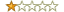Join Date: Jan 2010 Posts: 60 Rept. Given: 12 Rept. Rcvd 32 Times in 14 Posts Thanks Given: 35 Thanks Rcvd at 74 Times in 20 Posts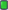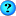Strange string encoding in C code

Can someone explain how this encoding works, thanks

Code:
```int a[]={21,19,6,17,12};
for(int i=0;i<5;++i){
int c=0;
for(int j=0;j<5;++j){
c+=a[j]<<(i*j);
}
printf("%c",'a'+c%31);
}```
#2
 dilaFriendJoin Date: Jan 2010 Posts: 60 Rept. Given: 12 Rept. Rcvd 32 Times in 14 Posts Thanks Given: 35 Thanks Rcvd at 74 Times in 20 PostsThis is interesting to me because it would lead to a nice key extraction challenge where the output is compared to a fixed string, then the objective is to figure out the list of magic numbers that encode to that string.

For example, if the output is "ninja", then the solution key is {21,19,6,17,12}, as it is in the example code i pasted.

Can anyone derive a set of coefficients that decode to a text string of their choice using the above code?
#3
 evlncrn8VIP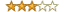Join Date: Sep 2005 Posts: 178 Rept. Given: 36 Rept. Rcvd 54 Times in 24 Posts Thanks Given: 47 Thanks Rcvd at 119 Times in 70 Postsim not so sure but is the 5 in the 'example' the length of the data ? in which case, you could just do the inverse, make a function taking a char* and instead of the << change it to >> and print the hex of the char, i think that'd work
#4
 dilaFriendJoin Date: Jan 2010 Posts: 60 Rept. Given: 12 Rept. Rcvd 32 Times in 14 Posts Thanks Given: 35 Thanks Rcvd at 74 Times in 20 PostsI created a key extraction challenge using this method. Maybe it can be solved?

Code:
```bool check(const std::string& key) {
uint64_t b = 0;
for(size_t i = 0; i < key.length(); ++i) {
uint64_t a = 0;
for(size_t j = 0; j < key.length(); ++j) {
uint64_t d=uint64_t(key[j]);
a += uint64_t(key[j]) << (i * j);
}
b += (a % 127) << (i * 8);
}
return (b == 0x000b762957335657);
}```
I don't think shifting in the opposite direction will work though @evlncrn8
#5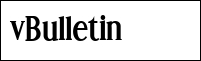deepzeroVIPJoin Date: Mar 2010 Location: Germany Posts: 283 Rept. Given: 104 Rept. Rcvd 62 Times in 40 Posts Thanks Given: 132 Thanks Rcvd at 177 Times in 83 PostsLooks like a simple system of equations with << operator.
 The Following User Says Thank You to deepzero For This Useful Post: dila (02-09-2018)
#6UniSoftFamily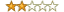Join Date: May 2010 Location: Shenzhen, China Posts: 118 Rept. Given: 22 Rept. Rcvd 252 Times in 40 Posts Thanks Given: 18 Thanks Rcvd at 364 Times in 68 PostsQuote:
 Originally Posted by dilaI created a key extraction challenge using this method. Maybe it can be solved? Code: ```bool check(const std::string& key) { uint64_t b = 0; for(size_t i = 0; i < key.length(); ++i) { uint64_t a = 0; for(size_t j = 0; j < key.length(); ++j) { uint64_t d=uint64_t(key[j]); a += uint64_t(key[j]) << (i * j); } b += (a % 127) << (i * 8); } return (b == 0x000b762957335657); }``` I don't think shifting in the opposite direction will work though @evlncrn8
I don't think that this can be solved in opposite direction...
I think only simple brootforce, maximum 7 characters (2nd-8th), 1st character you calculate like ([sum of all characters] % 127) == (b & 0x7F))
 The Following User Says Thank You to UniSoft For This Useful Post: dila (02-09-2018)
#7
 dilaFriendJoin Date: Jan 2010 Posts: 60 Rept. Given: 12 Rept. Rcvd 32 Times in 14 Posts Thanks Given: 35 Thanks Rcvd at 74 Times in 20 PostsThank you for trying. Yes, it can be solved and @deepzero has the right idea. It is a linear system of equations. As a hint, try replacing the shifting with a multiplication by powers-of-two, so x << y becomes x*2^y, then you might see the solution#8
 dilaFriendJoin Date: Jan 2010 Posts: 60 Rept. Given: 12 Rept. Rcvd 32 Times in 14 Posts Thanks Given: 35 Thanks Rcvd at 74 Times in 20 PostsSome more hints:

* Write it as a matrix multiplication
* See that 127 is a mersenne prime (2^7-1) and that this makes makes modular arithmetic operations in a finite field.
#9
 floatersFriendJoin Date: Apr 2018 Posts: 8 Rept. Given: 0 Rept. Rcvd 1 Time in 1 Post Thanks Given: 6 Thanks Rcvd at 6 Times in 3 PostsInteresting puzzleThe solution is to write the problem as a matrix vector multiplication and invert the matrix using integer modulo arithmetic. The key is then given by multiplying with the inverse matrix with modulo.

For the first problem of key length 5 and modulo 31 the inverse matrix is
{
{25, 25, 25, 25, 25},
{25, 28, 14, 7, 19},
{25, 14, 19, 28, 7},
{25, 7, 28, 19, 14},
{25, 19, 7, 14, 28}
}
For the problem of key length 7 and modulo 127 the inverse is:
{
{109, 109, 109, 109, 109, 109, 109},
{109, 118, 59, 93, 110, 55, 91},
{109, 59, 110, 91, 118, 93, 55},
{109, 93, 91, 59, 55, 118, 110},
{109, 110, 118, 55, 59, 91, 93},
{109, 55, 93, 118, 91, 110, 59},
{109, 91, 55, 110, 93, 59, 118}
}

i<3Code
 The Following User Gave Reputation+1 to floaters For This Useful Post: deepzero (04-04-2018)
 The Following 2 Users Say Thank You to floaters For This Useful Post: dila (04-09-2018), niculaita (04-05-2018)
#10
 dilaFriendJoin Date: Jan 2010 Posts: 60 Rept. Given: 12 Rept. Rcvd 32 Times in 14 Posts Thanks Given: 35 Thanks Rcvd at 74 Times in 20 PostsNice solution! As a final note, the problem was derived from a Fourier transform over the finite field. Note the 2^(i*j) factor, which is the finite equivalent of the complex Fourier transform exp(-2*pi*i*x*f) coefficient.

I noticed, while working on this, that the second row of this DFT matrix consists of all the powers-of-two and by multiplying this matrix by a vector we get an inner product:

<(a_0, a_1, a_2, ..., a_n), (2^0, 2^1, 2^2, ..., 2^n)>

Or in expanded form:

a_0 + a_1*2 + a_2*4 + a_3*8 + a_4*16 + a_5*32 + ...

Which is exactly the formula for conversion from binary to integer. In other words - in the Fourier transform of a binary vector over an appropriately chosen finite field, we get the integer representation of that binary number appear in the spectrum.

A quick demonstration:

The binary representation of 45 is

0 1 0 1 1 0 1

Now compute the Fourier transform over F_127 to obtain the spectrum vector:

4 45 89 71 99 27 53

Notice also that the "DC component" is 4, which is the hamming weight of the input binary vector (the number of 1's in the binary string).

Since the matrix is invertible (as described by floaters), we can also go back to the binary representation with by the inverse transform. Note that this only works if all the spectrum values are correct.

Here is a list of all binary vectors, and their spectrum, over F_127:

F{0 0 0 0 0 0 0} = {0 0 0 0 0 0 0}
F{1 0 0 0 0 0 0} = {1 1 1 1 1 1 1}
F{0 1 0 0 0 0 0} = {1 2 4 8 16 32 64}
F{1 1 0 0 0 0 0} = {2 3 5 9 17 33 65}
F{0 0 1 0 0 0 0} = {1 4 16 64 2 8 32}
F{1 0 1 0 0 0 0} = {2 5 17 65 3 9 33}
F{0 1 1 0 0 0 0} = {2 6 20 72 18 40 96}
F{1 1 1 0 0 0 0} = {3 7 21 73 19 41 97}
F{0 0 0 1 0 0 0} = {1 8 64 4 32 2 16}
F{1 0 0 1 0 0 0} = {2 9 65 5 33 3 17}
F{0 1 0 1 0 0 0} = {2 10 68 12 48 34 80}
F{1 1 0 1 0 0 0} = {3 11 69 13 49 35 81}
F{0 0 1 1 0 0 0} = {2 12 80 68 34 10 48}
F{1 0 1 1 0 0 0} = {3 13 81 69 35 11 49}
F{0 1 1 1 0 0 0} = {3 14 84 76 50 42 112}
F{1 1 1 1 0 0 0} = {4 15 85 77 51 43 113}
F{0 0 0 0 1 0 0} = {1 16 2 32 4 64 8}
F{1 0 0 0 1 0 0} = {2 17 3 33 5 65 9}
F{0 1 0 0 1 0 0} = {2 18 6 40 20 96 72}
F{1 1 0 0 1 0 0} = {3 19 7 41 21 97 73}
F{0 0 1 0 1 0 0} = {2 20 18 96 6 72 40}
F{1 0 1 0 1 0 0} = {3 21 19 97 7 73 41}
F{0 1 1 0 1 0 0} = {3 22 22 104 22 104 104}
F{1 1 1 0 1 0 0} = {4 23 23 105 23 105 105}
F{0 0 0 1 1 0 0} = {2 24 66 36 36 66 24}
F{1 0 0 1 1 0 0} = {3 25 67 37 37 67 25}
F{0 1 0 1 1 0 0} = {3 26 70 44 52 98 88}
F{1 1 0 1 1 0 0} = {4 27 71 45 53 99 89}
F{0 0 1 1 1 0 0} = {3 28 82 100 38 74 56}
F{1 0 1 1 1 0 0} = {4 29 83 101 39 75 57}
F{0 1 1 1 1 0 0} = {4 30 86 108 54 106 120}
F{1 1 1 1 1 0 0} = {5 31 87 109 55 107 121}
F{0 0 0 0 0 1 0} = {1 32 8 2 64 16 4}
F{1 0 0 0 0 1 0} = {2 33 9 3 65 17 5}
F{0 1 0 0 0 1 0} = {2 34 12 10 80 48 68}
F{1 1 0 0 0 1 0} = {3 35 13 11 81 49 69}
F{0 0 1 0 0 1 0} = {2 36 24 66 66 24 36}
F{1 0 1 0 0 1 0} = {3 37 25 67 67 25 37}
F{0 1 1 0 0 1 0} = {3 38 28 74 82 56 100}
F{1 1 1 0 0 1 0} = {4 39 29 75 83 57 101}
F{0 0 0 1 0 1 0} = {2 40 72 6 96 18 20}
F{1 0 0 1 0 1 0} = {3 41 73 7 97 19 21}
F{0 1 0 1 0 1 0} = {3 42 76 14 112 50 84}
F{1 1 0 1 0 1 0} = {4 43 77 15 113 51 85}
F{0 0 1 1 0 1 0} = {3 44 88 70 98 26 52}
F{1 0 1 1 0 1 0} = {4 45 89 71 99 27 53}
F{0 1 1 1 0 1 0} = {4 46 92 78 114 58 116}
F{1 1 1 1 0 1 0} = {5 47 93 79 115 59 117}
F{0 0 0 0 1 1 0} = {2 48 10 34 68 80 12}
F{1 0 0 0 1 1 0} = {3 49 11 35 69 81 13}
F{0 1 0 0 1 1 0} = {3 50 14 42 84 112 76}
F{1 1 0 0 1 1 0} = {4 51 15 43 85 113 77}
F{0 0 1 0 1 1 0} = {3 52 26 98 70 88 44}
F{1 0 1 0 1 1 0} = {4 53 27 99 71 89 45}
F{0 1 1 0 1 1 0} = {4 54 30 106 86 120 108}
F{1 1 1 0 1 1 0} = {5 55 31 107 87 121 109}
F{0 0 0 1 1 1 0} = {3 56 74 38 100 82 28}
F{1 0 0 1 1 1 0} = {4 57 75 39 101 83 29}
F{0 1 0 1 1 1 0} = {4 58 78 46 116 114 92}
F{1 1 0 1 1 1 0} = {5 59 79 47 117 115 93}
F{0 0 1 1 1 1 0} = {4 60 90 102 102 90 60}
F{1 0 1 1 1 1 0} = {5 61 91 103 103 91 61}
F{0 1 1 1 1 1 0} = {5 62 94 110 118 122 124}
F{1 1 1 1 1 1 0} = {6 63 95 111 119 123 125}
F{0 0 0 0 0 0 1} = {1 64 32 16 8 4 2}
F{1 0 0 0 0 0 1} = {2 65 33 17 9 5 3}
F{0 1 0 0 0 0 1} = {2 66 36 24 24 36 66}
F{1 1 0 0 0 0 1} = {3 67 37 25 25 37 67}
F{0 0 1 0 0 0 1} = {2 68 48 80 10 12 34}
F{1 0 1 0 0 0 1} = {3 69 49 81 11 13 35}
F{0 1 1 0 0 0 1} = {3 70 52 88 26 44 98}
F{1 1 1 0 0 0 1} = {4 71 53 89 27 45 99}
F{0 0 0 1 0 0 1} = {2 72 96 20 40 6 18}
F{1 0 0 1 0 0 1} = {3 73 97 21 41 7 19}
F{0 1 0 1 0 0 1} = {3 74 100 28 56 38 82}
F{1 1 0 1 0 0 1} = {4 75 101 29 57 39 83}
F{0 0 1 1 0 0 1} = {3 76 112 84 42 14 50}
F{1 0 1 1 0 0 1} = {4 77 113 85 43 15 51}
F{0 1 1 1 0 0 1} = {4 78 116 92 58 46 114}
F{1 1 1 1 0 0 1} = {5 79 117 93 59 47 115}
F{0 0 0 0 1 0 1} = {2 80 34 48 12 68 10}
F{1 0 0 0 1 0 1} = {3 81 35 49 13 69 11}
F{0 1 0 0 1 0 1} = {3 82 38 56 28 100 74}
F{1 1 0 0 1 0 1} = {4 83 39 57 29 101 75}
F{0 0 1 0 1 0 1} = {3 84 50 112 14 76 42}
F{1 0 1 0 1 0 1} = {4 85 51 113 15 77 43}
F{0 1 1 0 1 0 1} = {4 86 54 120 30 108 106}
F{1 1 1 0 1 0 1} = {5 87 55 121 31 109 107}
F{0 0 0 1 1 0 1} = {3 88 98 52 44 70 26}
F{1 0 0 1 1 0 1} = {4 89 99 53 45 71 27}
F{0 1 0 1 1 0 1} = {4 90 102 60 60 102 90}
F{1 1 0 1 1 0 1} = {5 91 103 61 61 103 91}
F{0 0 1 1 1 0 1} = {4 92 114 116 46 78 58}
F{1 0 1 1 1 0 1} = {5 93 115 117 47 79 59}
F{0 1 1 1 1 0 1} = {5 94 118 124 62 110 122}
F{1 1 1 1 1 0 1} = {6 95 119 125 63 111 123}
F{0 0 0 0 0 1 1} = {2 96 40 18 72 20 6}
F{1 0 0 0 0 1 1} = {3 97 41 19 73 21 7}
F{0 1 0 0 0 1 1} = {3 98 44 26 88 52 70}
F{1 1 0 0 0 1 1} = {4 99 45 27 89 53 71}
F{0 0 1 0 0 1 1} = {3 100 56 82 74 28 38}
F{1 0 1 0 0 1 1} = {4 101 57 83 75 29 39}
F{0 1 1 0 0 1 1} = {4 102 60 90 90 60 102}
F{1 1 1 0 0 1 1} = {5 103 61 91 91 61 103}
F{0 0 0 1 0 1 1} = {3 104 104 22 104 22 22}
F{1 0 0 1 0 1 1} = {4 105 105 23 105 23 23}
F{0 1 0 1 0 1 1} = {4 106 108 30 120 54 86}
F{1 1 0 1 0 1 1} = {5 107 109 31 121 55 87}
F{0 0 1 1 0 1 1} = {4 108 120 86 106 30 54}
F{1 0 1 1 0 1 1} = {5 109 121 87 107 31 55}
F{0 1 1 1 0 1 1} = {5 110 124 94 122 62 118}
F{1 1 1 1 0 1 1} = {6 111 125 95 123 63 119}
F{0 0 0 0 1 1 1} = {3 112 42 50 76 84 14}
F{1 0 0 0 1 1 1} = {4 113 43 51 77 85 15}
F{0 1 0 0 1 1 1} = {4 114 46 58 92 116 78}
F{1 1 0 0 1 1 1} = {5 115 47 59 93 117 79}
F{0 0 1 0 1 1 1} = {4 116 58 114 78 92 46}
F{1 0 1 0 1 1 1} = {5 117 59 115 79 93 47}
F{0 1 1 0 1 1 1} = {5 118 62 122 94 124 110}
F{1 1 1 0 1 1 1} = {6 119 63 123 95 125 111}
F{0 0 0 1 1 1 1} = {4 120 106 54 108 86 30}
F{1 0 0 1 1 1 1} = {5 121 107 55 109 87 31}
F{0 1 0 1 1 1 1} = {5 122 110 62 124 118 94}
F{1 1 0 1 1 1 1} = {6 123 111 63 125 119 95}
F{0 0 1 1 1 1 1} = {5 124 122 118 110 94 62}
F{1 0 1 1 1 1 1} = {6 125 123 119 111 95 63}
F{0 1 1 1 1 1 1} = {6 126 126 126 126 126 126}
F{1 1 1 1 1 1 1} = {7 0 0 0 0 0 0}
 The Following User Gave Reputation+1 to dila For This Useful Post: deepzero (04-09-2018)
 The Following 2 Users Say Thank You to dila For This Useful Post: deepzero (04-09-2018), floaters (04-10-2018)
#11
 floatersFriendJoin Date: Apr 2018 Posts: 8 Rept. Given: 0 Rept. Rcvd 1 Time in 1 Post Thanks Given: 6 Thanks Rcvd at 6 Times in 3 PostsOh cool, I've never looked at the DFT in finite fields before. Looking at it from the DFT perspective, it means that we can compute the inverse DFT directly without having to invert the matrix.

The inverse DFT is given by N^(-1) * {{a^(- i * j)}},
and since we work in the finite field over 127, the negative exponent is resolved
by modular inverse in 127:

ModInverse(N, 127) * {{ModInverse(2^(i * j), 127)}}

i,j are the matrix indices from 0 to N-1.
Here N=7 and ModInverse(7, 127) = 105 for example.

That makes computing the inverse for any such invertible problem much easier!
 The Following User Says Thank You to floaters For This Useful Post: dila (04-10-2018)

 Thread ToolsShow Printable VersionEmail this Page Display ModesLinear ModeSwitch to Hybrid ModeSwitch to Threaded ModePosting Rules You may not post new threads You may not post replies You may not post attachments You may not edit your posts BB code is On Smilies are On [IMG] code is On HTML code is On Forum RulesSimilar Threads Thread Thread Starter Forum Replies Last Post TempoMat General Discussion 11 03-29-2020 17:58 XorRanger Source Code 0 04-30-2015 15:37

All times are GMT +8. The time now is 09:02.

 Aaron's homepage - TopAlways Your Best Friend: Aaron, JMI, ahmadmansoor, ZeNiX
( 1998 - 2021 )